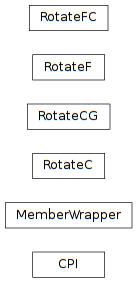# 5.1. pyopus.problems.cpi — Common problem interface¶Common problem interface (PyOPUS subsystem name: CPI)

Common problem interface (CPI) is a picklable dictionary containing all the information that is needed to describe an optimization problem.

The dictionary contains the following members:

• setup – Setup function. If not None this function must be called just before the problem is evaluated for the first time.
• name – problem name
• n – number of variables
• m – number of nonlinear constraints
• x0 – the initial point
• f – returns the cost function value
• g – returns the cost function gradient
• c – returns the vector of nonlinear constraints
• cg – returns the Jacobian of the constraint functions, one row corresponds to one constraint, columns represent variables
• fc – returns the cost function value and the constraint function values
• xlo – a vector of lower bounds on variables
• xhi – a vector of upper bounds on variables
• clo – a vector of lower bounds on nonlinear constraints
• chi – a vector of upper bounds on nonlinear constraints
• fmin – best known minimum function value
• xmin – best known minimum
• info – additional problem information dictionary (suite dependent)

Lower and upper bounds are always defined. If some bound is not defined, it is set to +Inf or -Inf. If some memeber is not available it is set to None.

If the setup member is not None the problem belongs to a suite where multiple problems share internal variables. For such problems the setup function should be called just before the first problem evaluation. If later the setup function of a different problem from the same suite is called the values of the shared internal variables change and all subsequent evaluations of the previous problem result in incorrect values.

This class is inherited by optimization problems that support CPI. The cpi() method returns the CPI dictionary of the problem.

This module is independent of PyOPUS, meaning that it can be taken as is and used as a module in some other package.

Classes that inherit this class must reimplement the cpi() method.

class pyopus.problems.cpi.MemberWrapper(obj, memberName)

Wraps a member function in a callable object.

obj is the object and memberName is the name of the member to wrap.

pyopus.problems.cpi.rotateProblem(itf, Q, extremeBarrierBounds=False)

Rotate a problem described by itf using orthogonal matrix Q.

Feeds all functions with Qx instead of x.

Converts bounds to constraints.

Treats rotated bounds with extreme barrier approach if extremeBarrierBounds is True.

#### Previous topic

5. pyopus.problems — Test problems for optimizaion algorithms

#### Next topic

5.2. pyopus.problems.glbc — Global optimization test functions